# 3.3. 模型评估: 量化预测的质量

## 3.3.1. scoring 参数: 定义模型评估规则

Model selection （模型选择）和 evaluation （评估）使用工具，例如 model_selection.GridSearchCVmodel_selection.cross_val_score ，采用 scoring 参数来控制它们对 estimators evaluated （评估的估计量）应用的指标。

### 3.3.1.1. 常见场景: 预定义值

Scoring（得分） Function（函数） Comment（注解）
Classification（分类）
‘accuracy’ metrics.accuracy_score
‘average_precision’ metrics.average_precision_score
‘f1’ metrics.f1_score for binary targets（用于二进制目标）
‘f1_micro’ metrics.f1_score micro-averaged（微平均）
‘f1_macro’ metrics.f1_score macro-averaged（宏平均）
‘f1_weighted’ metrics.f1_score weighted average（加权平均）
‘f1_samples’ metrics.f1_score by multilabel sample（通过 multilabel 样本）
‘neg_log_loss’ metrics.log_loss requires predict_proba support（需要 predict_proba 支持）
‘precision’ etc. metrics.precision_score suffixes apply as with ‘f1’（后缀适用于 ‘f1’）
‘recall’ etc. metrics.recall_score suffixes apply as with ‘f1’（后缀适用于 ‘f1’）
‘roc_auc’ metrics.roc_auc_score
Clustering（聚类）
‘adjusted_mutual_info_score’ metrics.adjusted_mutual_info_score
‘adjusted_rand_score’ metrics.adjusted_rand_score
‘completeness_score’ metrics.completeness_score
‘fowlkes_mallows_score’ metrics.fowlkes_mallows_score
‘homogeneity_score’ metrics.homogeneity_score
‘mutual_info_score’ metrics.mutual_info_score
‘normalized_mutual_info_score’ metrics.normalized_mutual_info_score
‘v_measure_score’ metrics.v_measure_score
Regression（回归）
‘explained_variance’ metrics.explained_variance_score
‘neg_mean_absolute_error’ metrics.mean_absolute_error
‘neg_mean_squared_error’ metrics.mean_squared_error
‘neg_mean_squared_log_error’ metrics.mean_squared_log_error
‘neg_median_absolute_error’ metrics.median_absolute_error
‘r2’ metrics.r2_score

>>> from sklearn import svm, datasets
>>> from sklearn.model_selection import cross_val_score
>>> X, y = iris.data, iris.target
>>> clf = svm.SVC(probability=True, random_state=0)
>>> cross_val_score(clf, X, y, scoring='neg_log_loss')
array([-0.07..., -0.16..., -0.06...])
>>> model = svm.SVC()
>>> cross_val_score(model, X, y, scoring='wrong_choice')
Traceback (most recent call last):
ValueError: 'wrong_choice' is not a valid scoring value. Valid options are ['accuracy', 'adjusted_mutual_info_score', 'adjusted_rand_score', 'average_precision', 'completeness_score', 'explained_variance', 'f1', 'f1_macro', 'f1_micro', 'f1_samples', 'f1_weighted', 'fowlkes_mallows_score', 'homogeneity_score', 'mutual_info_score', 'neg_log_loss', 'neg_mean_absolute_error', 'neg_mean_squared_error', 'neg_mean_squared_log_error', 'neg_median_absolute_error', 'normalized_mutual_info_score', 'precision', 'precision_macro', 'precision_micro', 'precision_samples', 'precision_weighted', 'r2', 'recall', 'recall_macro', 'recall_micro', 'recall_samples', 'recall_weighted', 'roc_auc', 'v_measure_score']



• ValueError exception 列出的值对应于以下部分描述的 functions measuring prediction accuracy （测量预测精度的函数）。 这些函数的 scorer objects （记分对象）存储在 dictionary sklearn.metrics.SCORERS 中。

### 3.3.1.2. 根据 metric 函数定义您的评分策略

• 函数以 _score 结尾返回一个值来最大化，越高越好。
• 函数 _error_loss 结尾返回一个值来 minimize （最小化），越低越好。当使用 make_scorer 转换成 scorer object （记分对象）时，将 greater_is_better 参数设置为 False（默认为 True; 请参阅下面的参数说明）。

>>> from sklearn.metrics import fbeta_score, make_scorer
>>> ftwo_scorer = make_scorer(fbeta_score, beta=2)
>>> from sklearn.model_selection import GridSearchCV
>>> from sklearn.svm import LinearSVC
>>> grid = GridSearchCV(LinearSVC(), param_grid={'C': [1, 10]}, scoring=ftwo_scorer)


• 你要使用的 python 函数（在下面的示例中是 my_custom_loss_func
• python 函数是否返回一个分数 (greater_is_better=True, 默认值) 或者一个 loss （损失） (greater_is_better=False)。 如果是一个 loss （损失），scorer object （记分对象）的 python 函数的输出被 negated （否定），符合 cross validation convention （交叉验证约定），scorers 为更好的模型返回更高的值。
• 仅用于 classification metrics （分类指数）: 您提供的 python 函数是否需要连续的 continuous decision certainties （判断确定性）（needs_threshold=True）。默认值为 False 。
• 任何其他参数，如 beta 或者 labels 在 函数 f1_score

>>> import numpy as np
>>> def my_custom_loss_func(y_true, y_pred):
...     diff = np.abs(y_true - y_pred).max()
...     return np.log1p(diff)
...
>>> # score will negate the return value of my_custom_loss_func,
>>> # which will be np.log(2), 0.693, given the values for X
>>> # and y defined below.
>>> score = make_scorer(my_custom_loss_func, greater_is_better=False)
>>> X = [, ]
>>> y = [0, 1]
>>> from sklearn.dummy import DummyClassifier
>>> clf = DummyClassifier(strategy='most_frequent', random_state=0)
>>> clf = clf.fit(X, y)
>>> my_custom_loss_func(clf.predict(X), y)
0.69...
>>> score(clf, X, y)
-0.69...



### 3.3.1.3. 实现自己的记分对象

• 可以使用参数 (estimator, X, y) 来调用它，其中 estimator 是要被评估的模型，X 是验证数据， yX (在有监督情况下) 或 None (在无监督情况下) 已经被标注的真实数据目标。
• 它返回一个浮点数，用于对 X 进行量化 estimator 的预测质量，参考 y 。 再次，按照惯例，更高的数字更好，所以如果你的 scorer 返回 loss ，那么这个值应该被 negated 。

• 虽然在调用函数的旁边定义自定义计分函数应该使用默认的joblib后端(loky)，但是从另一个模块导入它将是一种更健壮的方法，并且独立于joblib后端。

• 例如，在下面的示例中，要使用大于1的n_jobs,custom_scoring_function函数保存在用户创建的模块中(custom_scorer_module.py)并导入:

>>> from custom_scorer_module import custom_scoring_function
>>> cross_val_score(model,
...  X_train,
...  y_train,
...  scoring=make_scorer(custom_scoring_function, greater_is_better=False),
...  cv=5,
...  n_jobs=-1)


### 3.3.1.4. 使用多个指数评估

Scikit-learn 还允许在 GridSearchCV, RandomizedSearchCVcross_validate 中评估 multiple metric （多个指数）。

scoring 参数指定多个评分指标有两种方法:

• 作为 string metrics 的迭代: py

scoring = ['accuracy', 'precision'] 

• 作为 dict，将 scorer 名称映射到 scoring 函数:

>>> from sklearn.metrics import accuracy_score
>>> from sklearn.metrics import make_scorer
>>> scoring = {'accuracy': make_scorer(accuracy_score),
...            'prec': 'precision'}


>>> from sklearn.model_selection import cross_validate
>>> from sklearn.metrics import confusion_matrix
>>> # A sample toy binary classification dataset
>>> X, y = datasets.make_classification(n_classes=2, random_state=0)
>>> svm = LinearSVC(random_state=0)
>>> def tn(y_true, y_pred): return confusion_matrix(y_true, y_pred)[0, 0]
>>> def fp(y_true, y_pred): return confusion_matrix(y_true, y_pred)[0, 1]
>>> def fn(y_true, y_pred): return confusion_matrix(y_true, y_pred)[1, 0]
>>> def tp(y_true, y_pred): return confusion_matrix(y_true, y_pred)[1, 1]
>>> scoring = {'tp': make_scorer(tp), 'tn': make_scorer(tn),
...            'fp': make_scorer(fp), 'fn': make_scorer(fn)}
>>> cv_results = cross_validate(svm.fit(X, y), X, y,
...                             scoring=scoring, cv=5)
>>> # Getting the test set true positive scores
>>> print(cv_results['test_tp'])
[10  9  8  7  8]
>>> # Getting the test set false negative scores
>>> print(cv_results['test_fn'])
[0 1 2 3 2]



## 3.3.2. 分类指标

sklearn.metrics 模块实现了几个 loss, score, 和 utility 函数来衡量 classification （分类）性能。 某些 metrics （指标）可能需要 positive class （正类），confidence values（置信度值）或 binary decisions values （二进制决策值）的概率估计。 大多数的实现允许每个样本通过 sample_weight 参数为 overall score （总分）提供 weighted contribution （加权贡献）。

precision_recall_curve(y_true, probas_pred) Compute precision-recall pairs for different probability thresholds
roc_curve(y_true, y_score[, pos_label, …]) Compute Receiver operating characteristic (ROC)

cohen_kappa_score(y1, y2[, labels, weights, …]) Cohen’s kappa: a statistic that measures inter-annotator agreement.
confusion_matrix(y_true, y_pred[, labels, …]) Compute confusion matrix to evaluate the accuracy of a classification
hinge_loss(y_true, pred_decision[, labels, …]) Average hinge loss (non-regularized)
matthews_corrcoef(y_true, y_pred[, …]) Compute the Matthews correlation coefficient (MCC)

accuracy_score(y_true, y_pred[, normalize, …]) Accuracy classification score.
classification_report(y_true, y_pred[, …]) Build a text report showing the main classification metrics
f1_score(y_true, y_pred[, labels, …]) Compute the F1 score, also known as balanced F-score or F-measure
fbeta_score(y_true, y_pred, beta[, labels, …]) Compute the F-beta score
hamming_loss(y_true, y_pred[, labels, …]) Compute the average Hamming loss.
jaccard_similarity_score(y_true, y_pred[, …]) Jaccard similarity coefficient score
log_loss(y_true, y_pred[, eps, normalize, …]) Log loss, aka logistic loss or cross-entropy loss.
precision_recall_fscore_support(y_true, y_pred) Compute precision, recall, F-measure and support for each class
precision_score(y_true, y_pred[, labels, …]) Compute the precision
recall_score(y_true, y_pred[, labels, …]) Compute the recall
zero_one_loss(y_true, y_pred[, normalize, …]) Zero-one classification loss.

dcg_score(y_true, y_score[, k]) Discounted cumulative gain (DCG) at rank K.
ndcg_score(y_true, y_score[, k]) Normalized discounted cumulative gain (NDCG) at rank K.

average_precision_score(y_true, y_score[, …]) Compute average precision (AP) from prediction scores
roc_auc_score(y_true, y_score[, average, …]) Compute Area Under the Curve (AUC) from prediction scores

### 3.3.2.1. 从二分到多分类和 multilabel

• "macro（宏）" 简单地计算 binary metrics （二分指标）的平均值，赋予每个类别相同的权重。在不常见的类别重要的问题上，macro-averaging （宏观平均）可能是突出表现的一种手段。另一方面，所有类别同样重要的假设通常是不真实的，因此 macro-averaging （宏观平均）将过度强调不频繁类的典型的低性能。
• "weighted（加权）" 通过计算其在真实数据样本中的存在来对每个类的 score 进行加权的 binary metrics （二分指标）的平均值来计算类不平衡。
• "micro（微）" 给每个 sample-class pair （样本类对）对 overall metric （总体指数）（sample-class 权重的结果除外） 等同的贡献。除了对每个类别的 metric 进行求和之外，这个总和构成每个类别度量的 dividends （除数）和 divisors （除数）计算一个整体商。 在 multilabel settings （多标签设置）中，Micro-averaging 可能是优先选择的，包括要忽略 majority class （多数类）的 multiclass classification （多类分类）。
• "samples（样本）" 仅适用于 multilabel problems （多标签问题）。它 does not calculate a per-class measure （不计算每个类别的 measure），而是计算 evaluation data （评估数据）中的每个样本的 true and predicted classes （真实和预测类别）的 metric （指标），并返回 (sample_weight-weighted) 加权平均。
• 选择 average=None 将返回一个 array 与每个类的 score 。

### 3.3.2.2. 精确度得分

accuracy_score 函数计算 accuracy, 正确预测的分数（默认）或计数 (normalize=False)。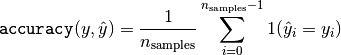>>> import numpy as np
>>> from sklearn.metrics import accuracy_score
>>> y_pred = [0, 2, 1, 3]
>>> y_true = [0, 1, 2, 3]
>>> accuracy_score(y_true, y_pred)
0.5
>>> accuracy_score(y_true, y_pred, normalize=False)
2



In the multilabel case with binary label indicators（在具有二分标签指示符的多标签情况下）:

>>> accuracy_score(np.array([[0, 1], [1, 1]]), np.ones((2, 2)))
0.5



### 3.3.2.3. Balanced accuracy score

balanced_accuracy_score函数计算 balanced accuracy, 它可以避免在不平衡数据集上作出夸大的性能估计。它是每个类的召回分数的宏观平均，或者，等价地，原始准确度，其中每个样本根据其真实类的样本占比率加权。因此，对均衡数据集，该函数的得分与准确率得分是相等的。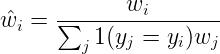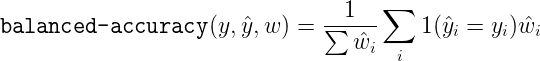• 我们定义:来自[Mosley2013], [Kelleher2015]和[Guyon2015], [Guyon2015]调整后的版本,以确保随机预测得分为0,完美的预测得分为1 .
• 如[Mosley2013]所述:计算每个类的精度与召回量之间的最小值。然后将这些值平均到类的总数上，以获得平衡的精度。
• 如[Urbanowicz2015]所述:计算每个类的敏感性和特异性的平均值，然后在总类数上取平均值。

### 3.3.2.4. Cohen’s kappa

kappa score （参阅 docstring ）是 -1 和 1 之间的数字。 .8 以上的 scores 通常被认为是很好的 agreement （协议）; 0 或者 更低表示没有 agreement （实际上是 random labels （随机标签））。

Kappa scores 可以计算 binary or multiclass （二分或者多分类）问题，但不能用于 multilabel problems （多标签问题）（除了手动计算 per-label score （每个标签分数）），而不是两个以上的 annotators （注释器）。

>>> from sklearn.metrics import cohen_kappa_score
>>> y_true = [2, 0, 2, 2, 0, 1]
>>> y_pred = [0, 0, 2, 2, 0, 2]
>>> cohen_kappa_score(y_true, y_pred)
0.4285714285714286



### 3.3.2.5. 混淆矩阵

confusion_matrix 函数通过计算 confusion matrix（混淆矩阵） 来 evaluates classification accuracy （评估分类的准确性）。

>>> from sklearn.metrics import confusion_matrix
>>> y_true = [2, 0, 2, 2, 0, 1]
>>> y_pred = [0, 0, 2, 2, 0, 2]
>>> confusion_matrix(y_true, y_pred)
array([[2, 0, 0],
[0, 0, 1],
[1, 0, 2]])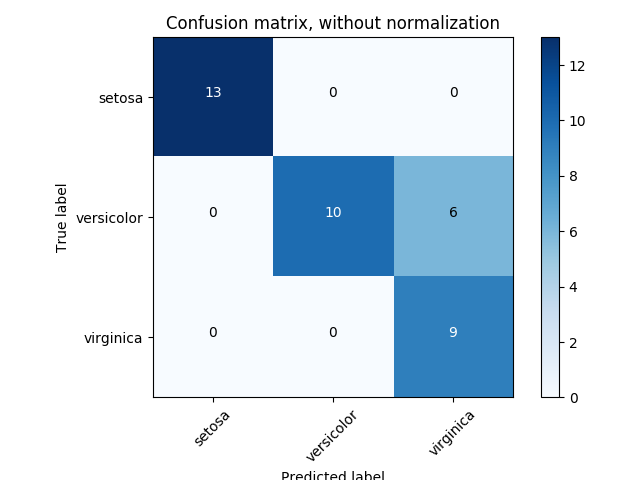>>> y_true = [0, 0, 0, 1, 1, 1, 1, 1]
>>> y_pred = [0, 1, 0, 1, 0, 1, 0, 1]
>>> tn, fp, fn, tp = confusion_matrix(y_true, y_pred).ravel()
>>> tn, fp, fn, tp
(2, 1, 2, 3)


### 3.3.2.6. 分类报告

classification_report 函数构建一个显示 main classification metrics （主分类指标）的文本报告。这是一个小示例，其中包含自定义的 target_names 和 inferred labels （推断标签）:

>>> from sklearn.metrics import classification_report
>>> y_true = [0, 1, 2, 2, 0]
>>> y_pred = [0, 0, 2, 1, 0]
>>> target_names = ['class 0', 'class 1', 'class 2']
>>> print(classification_report(y_true, y_pred, target_names=target_names))
precision    recall  f1-score   support

class 0       0.67      1.00      0.80         2
class 1       0.00      0.00      0.00         1
class 2       1.00      0.50      0.67         2

accuracy                           0.60         5
macro avg       0.56      0.50      0.49         5
weighted avg       0.67      0.60      0.59         5


### 3.3.2.7. 汉明损失

hamming_loss 计算两组样本之间的 average Hamming loss （平均汉明损失）或者 Hamming distance（汉明距离）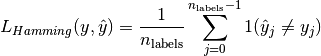>>> from sklearn.metrics import hamming_loss
>>> y_pred = [1, 2, 3, 4]
>>> y_true = [2, 2, 3, 4]
>>> hamming_loss(y_true, y_pred)
0.25



>>> hamming_loss(np.array([[0, 1], [1, 1]]), np.zeros((2, 2)))
0.75



• 在 multiclass classification （多类分类）中， Hamming loss （汉明损失）对应于 y_truey_pred 之间的 Hamming distance（汉明距离），它类似于 零一损失 函数。然而， zero-one loss penalizes （0-1损失惩罚）不严格匹配真实集合的预测集，Hamming loss （汉明损失）惩罚 individual labels （独立标签）。因此，Hamming loss（汉明损失）高于 zero-one loss（0-1 损失），总是在 0 和 1 之间，包括 0 和 1;预测真正的标签的正确的 subset or superset （子集或超集）将给出 0 和 1 之间的 Hamming loss（汉明损失）。

### 3.3.2.8. 精准，召回和 F-measures

F-measure (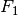measures) 可以解释为 precision （精度）和 recall （召回）的 weighted harmonic mean （加权调和平均值）。measure 值达到其最佳值 1 ，其最差分数为 0 。与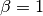,是等价的， recall （召回）和 precision （精度）同样重要。

precision_recall_curve 通过改变 decision threshold （决策阈值）从 ground truth label （被标记的真实数据标签） 和 score given by the classifier （分类器给出的分数）计算 precision-recall curve （精确召回曲线）。

average_precision_score 函数根据 prediction scores （预测分数）计算出 average precision (AP)（平均精度）。该分数对应于 precision-recall curve （精确召回曲线）下的面积。该值在 0 和 1 之间，并且越高越好。通过 random predictions （随机预测）， AP 是 fraction of positive samples （正样本的分数）。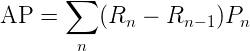average_precision_score(y_true, y_score[, …]) Compute average precision (AP) from prediction scores
f1_score(y_true, y_pred[, labels, …]) Compute the F1 score, also known as balanced F-score or F-measure
fbeta_score(y_true, y_pred, beta[, labels, …]) Compute the F-beta score
precision_recall_curve(y_true, probas_pred) Compute precision-recall pairs for different probability thresholds
precision_recall_fscore_support(y_true, y_pred) Compute precision, recall, F-measure and support for each class
precision_score(y_true, y_pred[, labels, …]) Compute the precision
recall_score(y_true, y_pred[, labels, …]) Compute the recall

#### 3.3.2.8.1. 二分类

Actual class (observation) Actual class (observation)
Predicted class (expectation) tp (true positive) Correct result fp (false positive) Unexpected result
Predicted class (expectation) fn (false negative) Missing result tn (true negative) Correct absence of result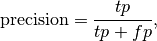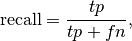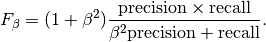>>> from sklearn import metrics
>>> y_pred = [0, 1, 0, 0]
>>> y_true = [0, 1, 0, 1]
>>> metrics.precision_score(y_true, y_pred)
1.0
>>> metrics.recall_score(y_true, y_pred)
0.5
>>> metrics.f1_score(y_true, y_pred)
0.66...
>>> metrics.fbeta_score(y_true, y_pred, beta=0.5)
0.83...
>>> metrics.fbeta_score(y_true, y_pred, beta=1)
0.66...
>>> metrics.fbeta_score(y_true, y_pred, beta=2)
0.55...
>>> metrics.precision_recall_fscore_support(y_true, y_pred, beta=0.5)
(array([0.66..., 1.        ]), array([1. , 0.5]), array([0.71..., 0.83...]), array([2, 2]))

>>> import numpy as np
>>> from sklearn.metrics import precision_recall_curve
>>> from sklearn.metrics import average_precision_score
>>> y_true = np.array([0, 0, 1, 1])
>>> y_scores = np.array([0.1, 0.4, 0.35, 0.8])
>>> precision, recall, threshold = precision_recall_curve(y_true, y_scores)
>>> precision
array([0.66..., 0.5       , 1.        , 1.        ])
>>> recall
array([1. , 0.5, 0.5, 0. ])
>>> threshold
array([0.35, 0.4 , 0.8 ])
>>> average_precision_score(y_true, y_scores)
0.83...


#### 3.3.2.8.2. 多类和多标签分类

•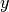predicted（预测）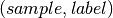•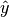true（真）•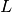labels 集合
•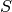samples 集合
•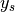的子集与样本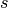, 即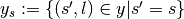•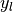的子集与 label• 类似的,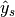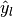的子集
•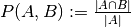•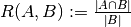(Conventions （公约）在处理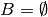有所不同; 这个实现使用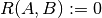, 与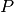类似.)
•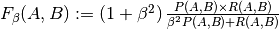average Precision Recall F_beta
"micro"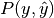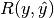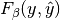"samples"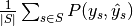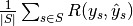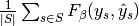"macro"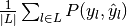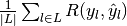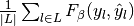"weighted"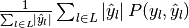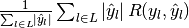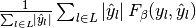None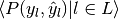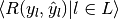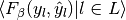>>> from sklearn import metrics
>>> y_true = [0, 1, 2, 0, 1, 2]
>>> y_pred = [0, 2, 1, 0, 0, 1]
>>> metrics.precision_score(y_true, y_pred, average='macro')
0.22...
>>> metrics.recall_score(y_true, y_pred, average='micro')
...
0.33...
>>> metrics.f1_score(y_true, y_pred, average='weighted')
0.26...
>>> metrics.fbeta_score(y_true, y_pred, average='macro', beta=0.5)
0.23...
>>> metrics.precision_recall_fscore_support(y_true, y_pred, beta=0.5, average=None)
...
(array([0.66..., 0.        , 0.        ]), array([1., 0., 0.]), array([0.71..., 0.        , 0.        ]), array([2, 2, 2]...))


For multiclass classification with a “negative class”, it is possible to exclude some labels:

>>> metrics.recall_score(y_true, y_pred, labels=[1, 2], average='micro')
... # excluding 0, no labels were correctly recalled
0.0



Similarly, labels not present in the data sample may be accounted for in macro-averaging.

>>> metrics.precision_score(y_true, y_pred, labels=[0, 1, 2, 3], average='macro')
...
0.166...



### 3.3.2.9. Jaccard 相似系数 score

jaccard_similarity_score 函数计算 pairs of label sets （标签组对）之间的 Jaccard similarity coefficients 也称作 Jaccard index 的平均值（默认）或总和。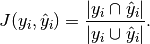jaccard_score就像precision_recall_fscore_support中的设定方法，本身应用于二分类，并通过使用从二分类扩展到多标记和多类（见从二分到多分类和multilabel）。

>>> import numpy as np
>>> from sklearn.metrics import jaccard_score
>>> y_true = np.array([[0, 1, 1],
...                    [1, 1, 0]])
>>> y_pred = np.array([[1, 1, 1],
...                    [1, 0, 0]])
>>> jaccard_score(y_true, y_pred)
0.6666...


>>> jaccard_score(y_true, y_pred, average='samples')
0.5833...
>>> jaccard_score(y_true, y_pred, average='macro')
0.6666...
>>> jaccard_score(y_true, y_pred, average=None)
array([0.5, 0.5, 1. ])


>>> y_pred = [0, 2, 1, 2]
>>> y_true = [0, 1, 2, 2]
>>> jaccard_score(y_true, y_pred, average=None)
...
array([1. , 0. , 0.33...])
>>> jaccard_score(y_true, y_pred, average='macro')
0.44...
>>> jaccard_score(y_true, y_pred, average='micro')
0.33...


### 3.3.2.10. Hinge loss

hinge_loss 函数使用 hinge loss 计算模型和数据之间的 average distance （平均距离），这是一种只考虑 prediction errors （预测误差）的 one-sided metric （单向指标）。（Hinge loss 用于最大边界分类器，如支持向量机）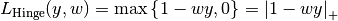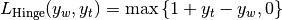>>> from sklearn import svm
>>> from sklearn.metrics import hinge_loss
>>> X = [, ]
>>> y = [-1, 1]
>>> est = svm.LinearSVC(random_state=0)
>>> est.fit(X, y)
LinearSVC(C=1.0, class_weight=None, dual=True, fit_intercept=True,
intercept_scaling=1, loss='squared_hinge', max_iter=1000,
multi_class='ovr', penalty='l2', random_state=0, tol=0.0001,
verbose=0)
>>> pred_decision = est.decision_function([[-2], , [0.5]])
>>> pred_decision
array([-2.18...,  2.36...,  0.09...])
>>> hinge_loss([-1, 1, 1], pred_decision)
0.3...



>>> X = np.array([, , , ])
>>> Y = np.array([0, 1, 2, 3])
>>> labels = np.array([0, 1, 2, 3])
>>> est = svm.LinearSVC()
>>> est.fit(X, Y)
LinearSVC(C=1.0, class_weight=None, dual=True, fit_intercept=True,
intercept_scaling=1, loss='squared_hinge', max_iter=1000,
multi_class='ovr', penalty='l2', random_state=None, tol=0.0001,
verbose=0)
>>> pred_decision = est.decision_function([[-1], , ])
>>> y_true = [0, 2, 3]
>>> hinge_loss(y_true, pred_decision, labels)
0.56...



### 3.3.2.11. Log 损失

Log loss，又被称为 logistic regression loss（logistic 回归损失）或者 cross-entropy loss（交叉熵损失） 定义在 probability estimates （概率估计）。它通常用于 (multinomial) logistic regression （（多项式）logistic 回归）和 neural networks （神经网络）以及 expectation-maximization （期望最大化）的一些变体中，并且可用于评估分类器的 probability outputs （概率输出）（predict_proba）而不是其 discrete predictions （离散预测）。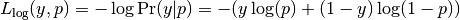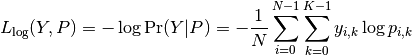log_loss 函数计算出一个 a list of ground-truth labels （已标注的真实数据的标签的列表）和一个 probability matrix （概率矩阵） 的 log loss，由 estimator （估计器）的 predict_proba 方法返回。

>>> from sklearn.metrics import log_loss
>>> y_true = [0, 0, 1, 1]
>>> y_pred = [[.9, .1], [.8, .2], [.3, .7], [.01, .99]]
>>> log_loss(y_true, y_pred)
0.1738...



y_pred 中的第一个 [.9, .1] 表示第一个样本具有标签 0 的 90% 概率。log loss 是非负数。

### 3.3.2.12. 马修斯相关系数

matthews_corrcoef 函数用于计算 binary classes （二分类）的 Matthew’s correlation coefficient (MCC) 引用自 Wikipedia:

“Matthews correlation coefficient（马修斯相关系数）用于机器学习，作为 binary (two-class) classifications （二分类）分类质量的度量。它考虑到 true and false positives and negatives （真和假的 positives 和 negatives），通常被认为是可以使用的 balanced measure（平衡措施），即使 classes are of very different sizes （类别大小不同）。MCC 本质上是 -1 和 +1 之间的相关系数值。系数 +1 表示完美预测，0 表示平均随机预测， -1 表示反向预测。statistic （统计量）也称为 phi coefficient （phi）系数。”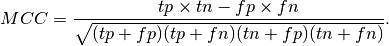•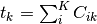真正发生了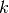类的次数,
•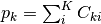类被预测的次数,
•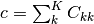正确预测的样本总数,
•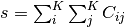样本总数.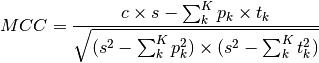>>> from sklearn.metrics import matthews_corrcoef
>>> y_true = [+1, +1, +1, -1]
>>> y_pred = [+1, -1, +1, +1]
>>> matthews_corrcoef(y_true, y_pred)
-0.33...



### 3.3.2.13. 多标记混淆矩阵

multilabel_confusion_matrix函数计算分类（默认）或样本（samplewise = True时）的多标记混淆矩阵，以评估分类的准确性。multilabel_confusion_matrix也将多类数据视为多标记数据，因为这是一种常用于评估二元分类指标（如精度，召回等）的多类问题的转换。

>>> import numpy as np
>>> from sklearn.metrics import multilabel_confusion_matrix
>>> y_true = np.array([[1, 0, 1],
...                    [0, 1, 0]])
>>> y_pred = np.array([[1, 0, 0],
...                    [0, 1, 1]])
>>> multilabel_confusion_matrix(y_true, y_pred)
array([[[1, 0],
[0, 1]],

[[1, 0],
[0, 1]],

[[0, 1],
[1, 0]]])


>>> multilabel_confusion_matrix(y_true, y_pred, samplewise=True)
array([[[1, 0],
[1, 1]],

[[1, 1],
[0, 1]]])


>>> y_true = ["cat", "ant", "cat", "cat", "ant", "bird"]
>>> y_pred = ["ant", "ant", "cat", "cat", "ant", "cat"]
>>> multilabel_confusion_matrix(y_true, y_pred,
...                             labels=["ant", "bird", "cat"])
array([[[3, 1],
[0, 2]],

[[5, 0],
[1, 0]],

[[2, 1],
[1, 2]]])


>>> y_true = np.array([[0, 0, 1],
...                    [0, 1, 0],
...                    [1, 1, 0]])
>>> y_pred = np.array([[0, 1, 0],
...                    [0, 0, 1],
...                    [1, 1, 0]])
>>> mcm = multilabel_confusion_matrix(y_true, y_pred)
>>> tn = mcm[:, 0, 0]
>>> tp = mcm[:, 1, 1]
>>> fn = mcm[:, 1, 0]
>>> fp = mcm[:, 0, 1]
>>> tp / (tp + fn)
array([1. , 0.5, 0. ])


>>> tn / (tn + fp)
array([1. , 0. , 0.5])


>>> fp / (fp + tn)
array([0. , 1. , 0.5])


>>> fn / (fn + tp)
array([0. , 0.5, 1. ])


### 3.3.2.14. Receiver operating characteristic (ROC)

“A receiver operating characteristic (ROC), 或者简单的 ROC 曲线，是一个图形图，说明了 binary classifier （二分分类器）系统的性能，因为 discrimination threshold （鉴别阈值）是变化的。它是通过在不同的阈值设置下，从 true positives out of the positives (TPR = true positive 比例) 与 false positives out of the negatives (FPR = false positive 比例) 绘制 true positive 的比例来创建的。 TPR 也称为 sensitivity（灵敏度），FPR 是减去 specificity（特异性） 或 true negative 比例。”

>>> import numpy as np
>>> from sklearn.metrics import roc_curve
>>> y = np.array([1, 1, 2, 2])
>>> scores = np.array([0.1, 0.4, 0.35, 0.8])
>>> fpr, tpr, thresholds = roc_curve(y, scores, pos_label=2)
>>> fpr
array([0. , 0. , 0.5, 0.5, 1. ])
>>> tpr
array([0. , 0.5, 0.5, 1. , 1. ])
>>> thresholds
array([1.8 , 0.8 , 0.4 , 0.35, 0.1 ])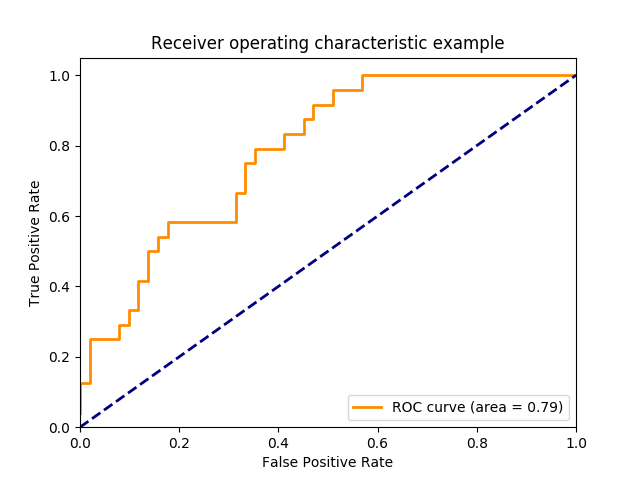roc_auc_score 函数计算 receiver operating characteristic (ROC) 曲线下的面积，也由 AUC 和 AUROC 表示。通过计算 roc 曲线下的面积，曲线信息总结为一个数字。 有关更多的信息，请参阅 Wikipedia article on AUC .

>>> import numpy as np
>>> from sklearn.metrics import roc_auc_score
>>> y_true = np.array([0, 0, 1, 1])
>>> y_scores = np.array([0.1, 0.4, 0.35, 0.8])
>>> roc_auc_score(y_true, y_scores)
0.75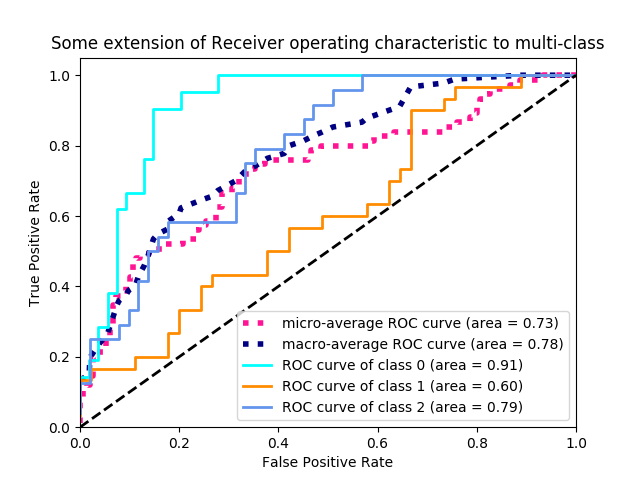### 3.3.2.15. 零一损失

zero_one_loss 函数通过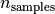计算 0-1 classification loss (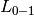) 的 sum （和）或 average （平均值）。默认情况下，函数在样本上 normalizes （标准化）。要获得的总和，将 normalize 设置为 False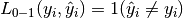>>> from sklearn.metrics import zero_one_loss
>>> y_pred = [1, 2, 3, 4]
>>> y_true = [2, 2, 3, 4]
>>> zero_one_loss(y_true, y_pred)
0.25
>>> zero_one_loss(y_true, y_pred, normalize=False)
1



>>> zero_one_loss(np.array([[0, 1], [1, 1]]), np.ones((2, 2)))
0.5

>>> zero_one_loss(np.array([[0, 1], [1, 1]]), np.ones((2, 2)),  normalize=False)
1



### 3.3.2.16. Brier 分数损失

brier_score_loss 函数计算二进制类的 Brier 分数 。引用维基百科：

“Brier 分数是一个特有的分数函数，用于衡量概率预测的准确性。它适用于预测必须将概率分配给一组相互排斥的离散结果的任务。”

Brier 分数损失也在0到1之间，分数越低（均方差越小），预测越准确。它可以被认为是对一组概率预测的 “校准” 的度量。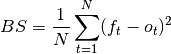>>> import numpy as np
>>> from sklearn.metrics import brier_score_loss
>>> y_true = np.array([0, 1, 1, 0])
>>> y_true_categorical = np.array(["spam", "ham", "ham", "spam"])
>>> y_prob = np.array([0.1, 0.9, 0.8, 0.4])
>>> y_pred = np.array([0, 1, 1, 0])
>>> brier_score_loss(y_true, y_prob)
0.055
>>> brier_score_loss(y_true, 1-y_prob, pos_label=0)
0.055
>>> brier_score_loss(y_true_categorical, y_prob, pos_label="ham")
0.055
>>> brier_score_loss(y_true, y_prob > 0.5)
0.0



## 3.3.3. 多标签排名指标

### 3.3.3.1. 覆盖误差

coverage_error 函数计算必须包含在最终预测中的标签的平均数，以便预测所有真正的标签。 如果您想知道有多少 top 评分标签，您必须通过平均来预测，而不会丢失任何真正的标签，这很有用。 因此，此指标的最佳价值是真正标签的平均数量。

• 我们的实现的得分比 Tsoumakas 等人在2010年的提出的计算方式大1。这扩展了它来处理一个具有0个真实标签实例的退化情况的能力。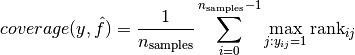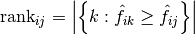。给定等级定义，通过给出将被分配给所有绑定值的最大等级， y_scores 中的关系会被破坏。

>>> import numpy as np
>>> from sklearn.metrics import coverage_error
>>> y_true = np.array([[1, 0, 0], [0, 0, 1]])
>>> y_score = np.array([[0.75, 0.5, 1], [1, 0.2, 0.1]])
>>> coverage_error(y_true, y_score)
2.5



### 3.3.3.2. 标签排名平均精度

label_ranking_average_precision_score 函数实现标签排名平均精度（LRAP）。 该度量值与 average_precision_score 函数相关联，但是基于标签排名的概念，而不是精确度和召回。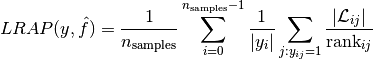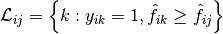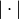是集合的 l0 范数或基数。

>>> import numpy as np
>>> from sklearn.metrics import label_ranking_average_precision_score
>>> y_true = np.array([[1, 0, 0], [0, 0, 1]])
>>> y_score = np.array([[0.75, 0.5, 1], [1, 0.2, 0.1]])
>>> label_ranking_average_precision_score(y_true, y_score)
0.416...



### 3.3.3.3. 排序损失

label_ranking_loss 函数计算在样本上平均排序错误的标签对数量的排序损失，即真实标签的分数低于假标签，由虚假和真实标签的倒数加权。最低可实现的排名损失为零。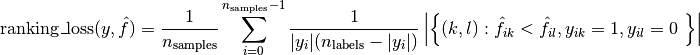>>> import numpy as np
>>> from sklearn.metrics import label_ranking_loss
>>> y_true = np.array([[1, 0, 0], [0, 0, 1]])
>>> y_score = np.array([[0.75, 0.5, 1], [1, 0.2, 0.1]])
>>> label_ranking_loss(y_true, y_score)
0.75...
>>> # With the following prediction, we have perfect and minimal loss
>>> y_score = np.array([[1.0, 0.1, 0.2], [0.1, 0.2, 0.9]])
>>> label_ranking_loss(y_true, y_score)
0.0



• Tsoumakas, G., Katakis, I., & Vlahavas, I. (2010). 挖掘多标签数据。在数据挖掘和知识发现手册（第667-685页）。美国 Springer.

## 3.3.4. 回归指标

sklearn.metrics 模块实现了一些 loss, score 以及 utility 函数以测量 regression（回归）的性能. 其中一些已经被加强以处理多个输出的场景: mean_squared_error, mean_absolute_error, explained_variance_scorer2_score.

r2_scoreexplained_variance_score 函数接受一个额外的值 'variance_weighted' 用于 multioutput 参数. 该选项通过相应目标变量的方差使得每个单独的 score 进行加权. 该设置量化了全局捕获的未缩放方差. 如果目标变量的大小不一样, 则该 score 更好地解释了较高的方差变量. multioutput='variance_weighted'r2_score 的默认值以向后兼容. 以后该值会被改成 uniform_average.

### 3.3.4.1. 解释方差得分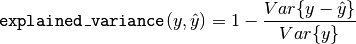>>> from sklearn.metrics import explained_variance_score
>>> y_true = [3, -0.5, 2, 7]
>>> y_pred = [2.5, 0.0, 2, 8]
>>> explained_variance_score(y_true, y_pred)
0.957...
>>> y_true = [[0.5, 1], [-1, 1], [7, -6]]
>>> y_pred = [[0, 2], [-1, 2], [8, -5]]
>>> explained_variance_score(y_true, y_pred, multioutput='raw_values')
...
array([ 0.967...,  1.        ])
>>> explained_variance_score(y_true, y_pred, multioutput=[0.3, 0.7])
...
0.990...



### 3.3.4.2. 最大误差

max_error函数计算最大残差，该度量捕获预测值和真实值之间的最坏情况误差。在完全拟合的单输出回归模型中，训练集上的max_error将为0，尽管在现实世界中这是极不可能的，但是这个度量显示了模型在拟合时的误差程度。

>>> from sklearn.metrics import max_error
>>> y_true = [3, 2, 7, 1]
>>> y_pred = [9, 2, 7, 1]
>>> max_error(y_true, y_pred)
6


max_error不支持多输出

### 3.3.4.3. 平均绝对误差

mean_absolute_error 函数计算了 平均绝对误差, 一个对应绝对误差损失预期值或者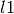-norm 损失的风险度量.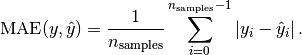>>> from sklearn.metrics import mean_absolute_error
>>> y_true = [3, -0.5, 2, 7]
>>> y_pred = [2.5, 0.0, 2, 8]
>>> mean_absolute_error(y_true, y_pred)
0.5
>>> y_true = [[0.5, 1], [-1, 1], [7, -6]]
>>> y_pred = [[0, 2], [-1, 2], [8, -5]]
>>> mean_absolute_error(y_true, y_pred)
0.75
>>> mean_absolute_error(y_true, y_pred, multioutput='raw_values')
array([ 0.5,  1. ])
>>> mean_absolute_error(y_true, y_pred, multioutput=[0.3, 0.7])
...
0.849...



### 3.3.4.4. 均方误差

mean_squared_error 函数计算了 均方误差, 一个对应于平方（二次）误差或损失的预期值的风险度量.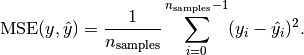>>> from sklearn.metrics import mean_squared_error
>>> y_true = [3, -0.5, 2, 7]
>>> y_pred = [2.5, 0.0, 2, 8]
>>> mean_squared_error(y_true, y_pred)
0.375
>>> y_true = [[0.5, 1], [-1, 1], [7, -6]]
>>> y_pred = [[0, 2], [-1, 2], [8, -5]]
>>> mean_squared_error(y_true, y_pred)
0.7083...



### 3.3.4.5. 均方误差对数

mean_squared_log_error 函数计算了一个对应平方对数（二次）误差或损失的预估值风险度量.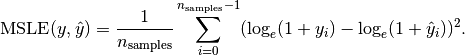>>> from sklearn.metrics import mean_squared_log_error
>>> y_true = [3, 5, 2.5, 7]
>>> y_pred = [2.5, 5, 4, 8]
>>> mean_squared_log_error(y_true, y_pred)
0.039...
>>> y_true = [[0.5, 1], [1, 2], [7, 6]]
>>> y_pred = [[0.5, 2], [1, 2.5], [8, 8]]
>>> mean_squared_log_error(y_true, y_pred)
0.044...



### 3.3.4.6. 中位绝对误差

median_absolute_error 函数尤其有趣, 因为它的离群值很强. 通过取目标和预测之间的所有绝对差值的中值来计算损失.median_absolute_error 函数不支持多输出.

>>> from sklearn.metrics import median_absolute_error
>>> y_true = [3, -0.5, 2, 7]
>>> y_pred = [2.5, 0.0, 2, 8]
>>> median_absolute_error(y_true, y_pred)
0.5



### 3.3.4.7. R² score, 可决系数

r2_score 函数计算了 computes R², 即 可决系数. 它提供了将来样本如何可能被模型预测的估量. 最佳分数为 1.0, 可以为负数（因为模型可能会更糟）. 总是预测 y 的预期值，不考虑输入特征的常数模型将得到 R^2 得分为 0.0.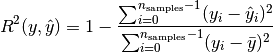>>> from sklearn.metrics import r2_score
>>> y_true = [3, -0.5, 2, 7]
>>> y_pred = [2.5, 0.0, 2, 8]
>>> r2_score(y_true, y_pred)
0.948...
>>> y_true = [[0.5, 1], [-1, 1], [7, -6]]
>>> y_pred = [[0, 2], [-1, 2], [8, -5]]
>>> r2_score(y_true, y_pred, multioutput='variance_weighted')
...
0.938...
>>> y_true = [[0.5, 1], [-1, 1], [7, -6]]
>>> y_pred = [[0, 2], [-1, 2], [8, -5]]
>>> r2_score(y_true, y_pred, multioutput='uniform_average')
...
0.936...
>>> r2_score(y_true, y_pred, multioutput='raw_values')
...
array([ 0.965...,  0.908...])
>>> r2_score(y_true, y_pred, multioutput=[0.3, 0.7])
...
0.925...



## 3.3.5. 聚类指标

sklearn.metrics 模块实现了一些 loss, score 和 utility 函数. 更多信息请参阅 聚类性能度量 部分, 例如聚类, 以及用于二分聚类的 Biclustering 评测.

## 3.3.6. 虚拟估计

• stratified 通过在训练集类分布方面来生成随机预测.
• most_frequent 总是预测训练集中最常见的标签.
• prior 总是给出能够最大化类先验概率的预测 (类似于 most_frequent) 并且 predict_proba 返回类先验概率.
• uniform 随机产生预测.
• constant总是预测用户提供的常量标签.当 positive class（正类）较少时,这种方法的主要动机是 F1-scoring.

>>> from sklearn.datasets import load_iris
>>> from sklearn.model_selection import train_test_split
>>> X, y = iris.data, iris.target
>>> y[y != 1] = -1
>>> X_train, X_test, y_train, y_test = train_test_split(X, y, random_state=0)


>>> from sklearn.dummy import DummyClassifier
>>> from sklearn.svm import SVC
>>> clf = SVC(kernel='linear', C=1).fit(X_train, y_train)
>>> clf.score(X_test, y_test)
0.63...
>>> clf = DummyClassifier(strategy='most_frequent',random_state=0)
>>> clf.fit(X_train, y_train)
DummyClassifier(constant=None, random_state=0, strategy='most_frequent')
>>> clf.score(X_test, y_test)
0.57...



>>> clf = SVC(gamma='scale', kernel='rbf', C=1).fit(X_train, y_train)
>>> clf.score(X_test, y_test)
0.94...



DummyRegressor 还实现了四个简单的经验法则来进行回归:

• mean 总是预测训练目标的平均值.
• median 总是预测训练目标的中位数.
• quantile 总是预测用户提供的训练目标的 quantile（分位数）.
• constant 总是预测由用户提供的常数值.